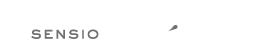# Rule `lowercase_cast`¶

Cast should be written in lower case.

## Examples¶

### Example #1¶

```--- Original
+++ New
<?php
-    \$a = (BOOLEAN) \$b;
-    \$a = (BOOL) \$b;
-    \$a = (INTEGER) \$b;
-    \$a = (INT) \$b;
-    \$a = (DOUBLE) \$b;
-    \$a = (FLoaT) \$b;
-    \$a = (reaL) \$b;
-    \$a = (flOAT) \$b;
-    \$a = (sTRING) \$b;
-    \$a = (ARRAy) \$b;
-    \$a = (OBJect) \$b;
-    \$a = (UNset) \$b;
-    \$a = (Binary) \$b;
+    \$a = (boolean) \$b;
+    \$a = (bool) \$b;
+    \$a = (integer) \$b;
+    \$a = (int) \$b;
+    \$a = (double) \$b;
+    \$a = (float) \$b;
+    \$a = (real) \$b;
+    \$a = (float) \$b;
+    \$a = (string) \$b;
+    \$a = (array) \$b;
+    \$a = (object) \$b;
+    \$a = (unset) \$b;
+    \$a = (binary) \$b;
```

### Example #2¶

```--- Original
+++ New
<?php
-    \$a = (BOOLEAN) \$b;
-    \$a = (BOOL) \$b;
-    \$a = (INTEGER) \$b;
-    \$a = (INT) \$b;
-    \$a = (DOUBLE) \$b;
-    \$a = (FLoaT) \$b;
-    \$a = (flOAT) \$b;
-    \$a = (sTRING) \$b;
-    \$a = (ARRAy) \$b;
-    \$a = (OBJect) \$b;
-    \$a = (UNset) \$b;
-    \$a = (Binary) \$b;
+    \$a = (boolean) \$b;
+    \$a = (bool) \$b;
+    \$a = (integer) \$b;
+    \$a = (int) \$b;
+    \$a = (double) \$b;
+    \$a = (float) \$b;
+    \$a = (float) \$b;
+    \$a = (string) \$b;
+    \$a = (array) \$b;
+    \$a = (object) \$b;
+    \$a = (unset) \$b;
+    \$a = (binary) \$b;
```

## Rule sets¶

The rule is part of the following rule sets:

@PER

Using the @PER rule set will enable the `lowercase_cast` rule.

@PSR12

Using the @PSR12 rule set will enable the `lowercase_cast` rule.

@PhpCsFixer

Using the @PhpCsFixer rule set will enable the `lowercase_cast` rule.

@Symfony

Using the @Symfony rule set will enable the `lowercase_cast` rule.

The logo is © 2010-2022 Sensio Labs